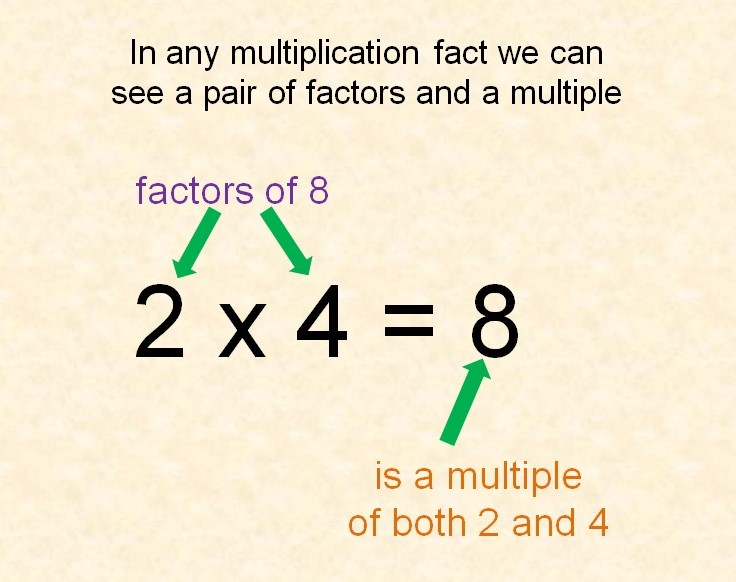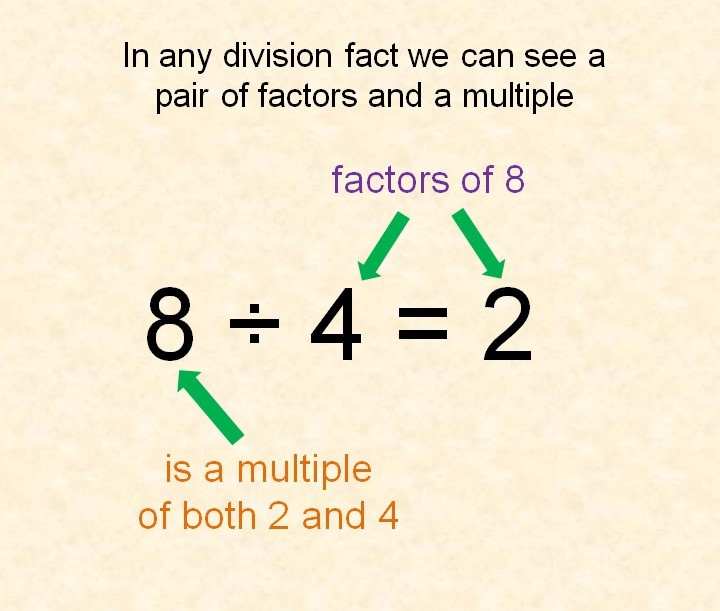## Multiples and factors

Multiples and factors are often confused because they are the inverse, or opposite, of each other.16 is a multiple of 8 because 8x2= 16

Using this fact, we also know that 8 and 2 are factors of 16, because the inverse division fact is 16÷ 8 = 2

We can look at any multiplication or division fact and identify a multiple and factors: